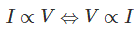## 6 Examples Of Ohm’s Law In Everyday Life

Ohm’s Law is the relationship between the current, voltage and resistance and which is derived by the German Physicist, George Simon Ohm. Ohm law’s application ranges from household appliances like heaters to the high tension wires and massive projects like rockets and spaceships.### Learn Physics in a fun way with Bright Spark App for free Now!

“Ohm law states that at a constant temperature, the current (I) flowing through the resistor is directly proportional to the voltage or potential difference (V) applied across the resistor”.The constant of proportionality is written as R and this is the resistance value of the resistor

V= RI

Let’s learn about its applications in our daily life activities.

### 1.  Conventional Domestic Fans

We can control the speed of the fans at our homes by moving the regulator to and fro. Here the current flowing through the fan is controlled by regulating the resistance through the regulator. A circular knob on the component can be rotated to achieve a variable resistance on the output terminals. For any specific value of the input, we can calculate the resistance, current and thus power flowing through Ohm’s Law.

### 2.  Electric Heaters

The electric heaters are the common appliances used in winters throughout the world. The heaters have a metal coil which has high resistance that permits a certain amount of current to flow through them to provide the required heat. Also, the power to be supplied to the heaters is calculated using this law.

### 3. Electric Kettles and Irons

The electric kettle and irons have a lot of resistors in them. The resistors limit the amount of current to flow through them to provide the required amount of heat. The size of resistors used in them is determined by using Ohm’s law.

### 4. Design of Electric Devices

The electronic devices such as laptop and mobile phones require a DC power supply with the specific current. Many devices need a certain amount of current and voltage to operate. Ohms law tells us the amount of resistance we need to establish a certain current with a certain amount of voltage.

### 5. Fuse Design

Fuses are the protection components that limit the amount of current flowing through the circuit and to establish a certain amount of voltage. They are connected in the series in the device. Ohms law is used to figure out which resistors are needed.

### 6. Mobile or Laptop Charger

Mobile and Laptop chargers use DC power supply in the operation. DC power supply provides a variable output voltage based on the resistance and the overall working is controlled by Ohm’s law.

Source

Share now: